Tổng cộng: 70Perimeter, area, surface area and volume

This animation presents the formulas to calculate the perimeter and area of shapes as...Solids of revolution

Rotating a geometric shape around a line within its geometric plane as an axis results in...Geometric transformations – reflection

This animation demonstrates geometric reflection, a type of geometric transformation both...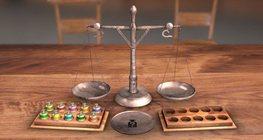Scale exercises

An interesting logical exercise: you have many weights that look the same, you have to...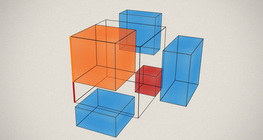Notable products

A spectacular demonstration of algebraic expressions.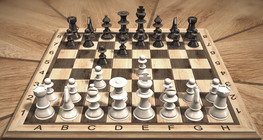Chess games

Chess games can help to develop logic skills.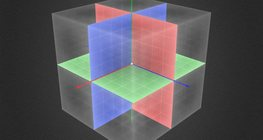Three-dimensional Cartesian coordinate system

3-dimensional Cartesian coordinate system with illustrations and exercises that develop...Geometric transformations – rotation

This animation demonstrates geometric rotation, a type of geometric transformation both...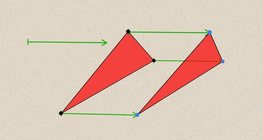Geometric transformations – translation

This animation demonstrates geometric translation in both plane and space.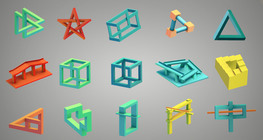Optical illusion

The information gathered by the eye is misinterpreted by the brain.Kepler´s laws of planetary motion

The three important laws describing planetary motion were formulated by Johannes Kepler.3D puzzle

This exciting and colourful game is designed to develop spatial perception. You can check...What is the time?

A game to practise the use of analogue and digital clocks.Ames room

An Ames room is a distorted room that is used to create an optical illusion.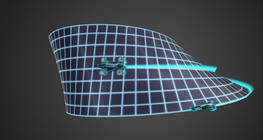Non-orientable surfaces

The Möbius strip and the Klein bottle are special two-dimensional surfaces with only one...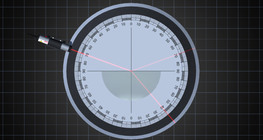The reflection and refraction of light

A ray of light is reflected or refracted at the boundary of two mediums with different...Dividing Space into Regions by 3 Planes

Division of space by three intersecting planes in several different arrangements.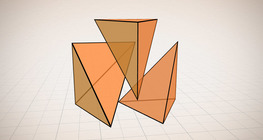Volume of a tetrahedron

To calculate the volume of a tetrahedron we start by calculating the volume of a prism.3D Tangram

A special, 3D version of the well-known Chinese logical game.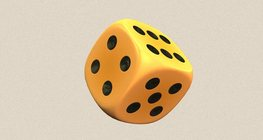Dice

Regular dice can be used for solving statistical and probability exercises.